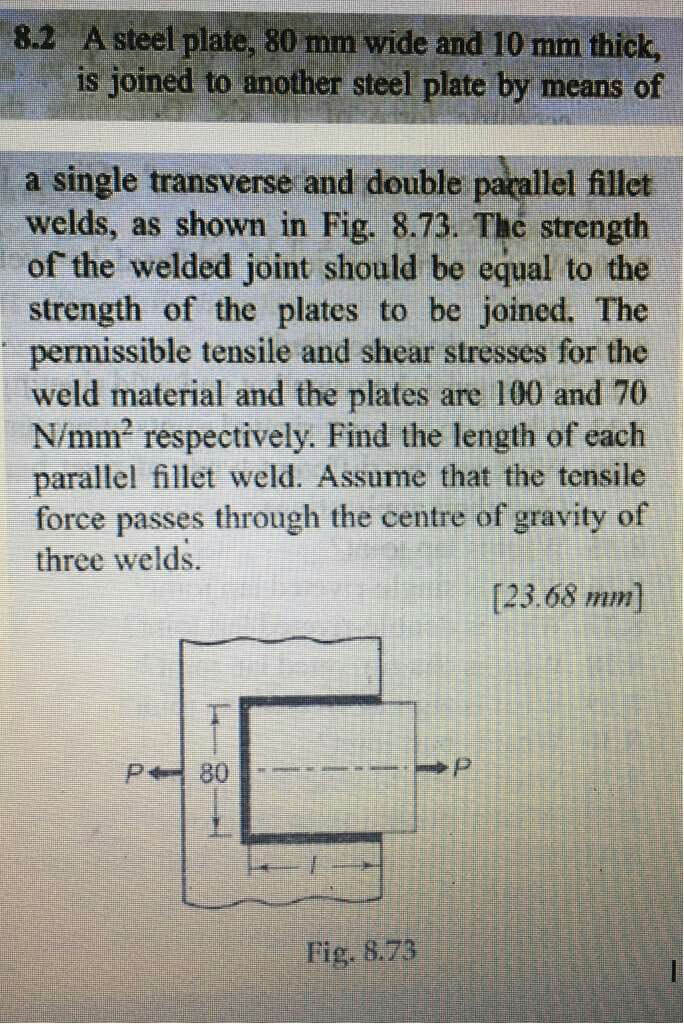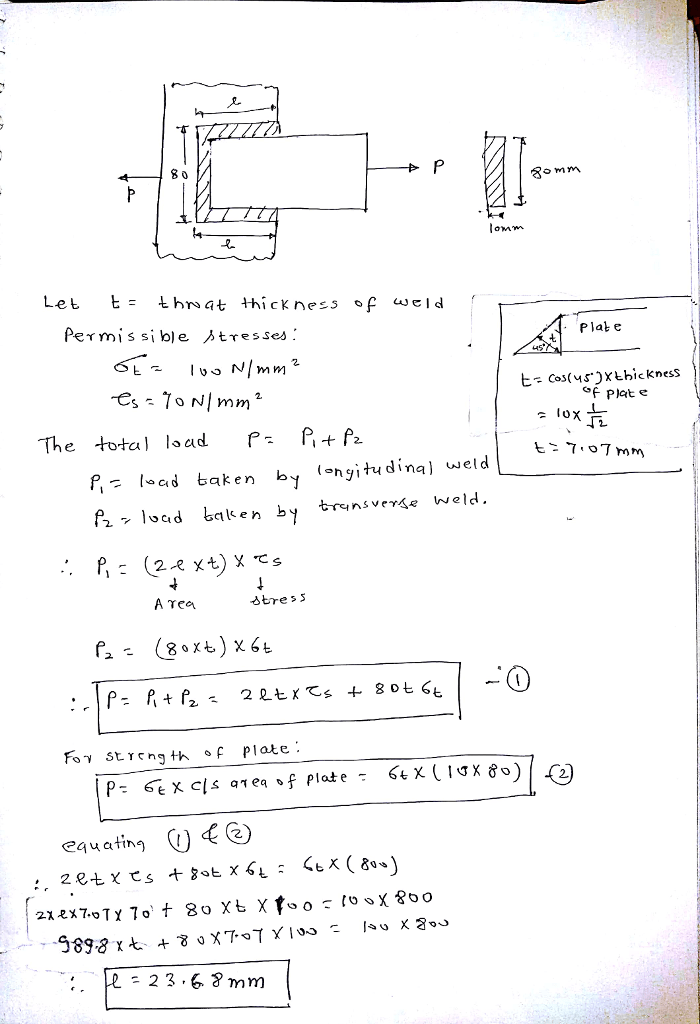# A steel plate, 80 mm wide and 10 mm thick is joined to another steel plate by means of a single transverse and double parallel fillet welds, as shown in Fig. 8.73. The strength of the welded joint should be equal to the strength of the plates to be joined. The permissible tensile and shear stresses for the weld material and the plates are 100 and 70 N/mm2 respectively. Find the length of each parallel fillet weld. Assume that the tensile force passes through the centre of gravity of three welds.

Question-AnswerCategory: Machine DesignA steel plate, 80 mm wide and 10 mm thick is joined to another steel plate by means of a single transverse and double parallel fillet welds, as shown in Fig. 8.73. The strength of the welded joint should be equal to the strength of the plates to be joined. The permissible tensile and shear stresses for the weld material and the plates are 100 and 70 N/mm2 respectively. Find the length of each parallel fillet weld. Assume that the tensile force passes through the centre of gravity of three welds.

A steel plate, 80 mm wide and 10 mm thick is joined to another steel plate by means of a single transverse and double parallel fillet welds, as shown in Fig. 8.73. The strength of the welded joint should be equal to the strength of the plates to be joined. The permissible tensile and shear stresses for the weld material and the plates are 100 and 70 N/mm2 respectively. Find the length of each parallel fillet weld. Assume that the tensile force passes through the centre of gravity of three welds.